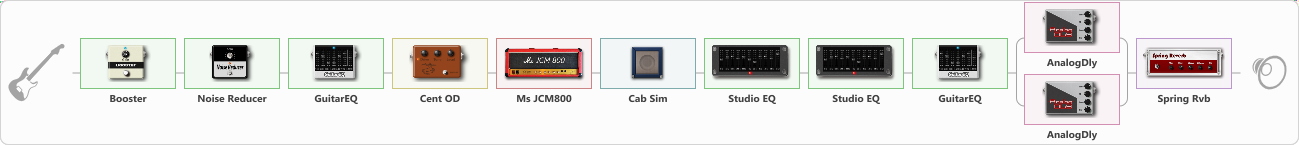Discussion in 'ToneLib-GFX presets' started by levitation, Apr 19, 2020.

1. Erik Zeal

I Just want to try this's vst, btw i'm very disappointed about the equalizer, in this vst I must use 4 equalizer just for find the character what i want, and tadaa.... the sound for me it's very nice

Effects chain:Effect: "Booster" (Dynamics / Filter), active - "yes"
{
"Gain" = 61
}

Effect: "Noise Reducer" (Dynamics / Filter), active - "yes"
{
"Sens" = 55
"Mode" = Soft
}

Effect: "GuitarEQ" (Dynamics / Filter), active - "yes"
{
"160 Hz" = 0
"400 Hz" = 0
"800 Hz" = 0
"1.6 kHz" = 0
"3.2 kHz" = 0
"6.4 kHz" = 9
"12 kHz" = 0
"Level (dB)" = 5
}

Effect: "Cent OD" (Overdrive / Distortion), active - "yes"
{
"Drive" = 13
"Tone" = 86
"Level" = 100
}

Effect: "Ms JCM800" (Amp simulators), active - "yes"
{
"Gain" = 63
"Bass" = 55
"Middle" = 46
"Treble" = 76
"Presence" = 32
"Master" = 52
"Level (dB)" = 9
}

Effect: "Cab Sim" (Cabinets), active - "yes"
{
"Model" = 4x12" Greenback
"Level (dB)" = 0
}

Effect: "Studio EQ" (Dynamics / Filter), active - "yes"
{
"31 Hz" = 0
"62 Hz" = 0
"125 Hz" = 0
"250 Hz" = 0
"500 Hz" = 1
"1 kHz" = -2
"2 kHz" = 0
"4 kHz" = -2
"8 kHz" = 2
"16 kHz" = 0
"Level (dB)" = 2
}

Effect: "Studio EQ" (Dynamics / Filter), active - "yes"
{
"31 Hz" = 0
"62 Hz" = 0
"125 Hz" = 0
"250 Hz" = 0
"500 Hz" = 0
"1 kHz" = 0
"2 kHz" = 0
"4 kHz" = -6
"8 kHz" = -7
"16 kHz" = -1
"Level (dB)" = -1
}

Effect: "GuitarEQ" (Dynamics / Filter), active - "yes"
{
"160 Hz" = 0
"400 Hz" = 0
"800 Hz" = 0
"1.6 kHz" = 0
"3.2 kHz" = 1
"6.4 kHz" = -6
"12 kHz" = 1
"Level (dB)" = -2
}

Effect: "Splitter" (Dynamics / Filter), active - "yes"
{
"A-Bypass" = Off
"A-Pan" = -49
"A-Level" = 55
"B-Bypass" = Off
"B-Pan" = 46
"B-Level" = 55

'A' branch:
{

Effect: "AnalogDly" (Delay), active - "yes"
{
"Time" = 380
"Feedback" = 48
"Tone" = 65
"Mix" = 48
}
}
'B' branch:
{

Effect: "AnalogDly" (Delay), active - "yes"
{
"Time" = 756
"Feedback" = 50
"Tone" = 65
"Mix" = 51
}
}
}

Effect: "Spring Rvb" (Reverb), active - "yes"
{
"Time" = 7.0
"PreDelay" = 0
"LoDamp" = 16
"HiDamp" = 26
"Mix" = 42
}

Note: You will need to download and install the ToneLib-GFX software to use the preset.

File size:
1.4 KB
Views:
2,868